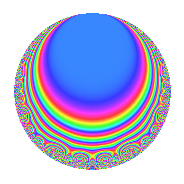# Properties

 Label 22.3.dLevel 22 Weight 3 Character orbit d Rep. character $$\chi_{22}(7,\cdot)$$ Character field $$\Q(\zeta_{10})$$ Dimension 8 Newforms 1 Sturm bound 9 Trace bound 0

# Related objects

## Defining parameters

 Level: $$N$$ = $$22 = 2 \cdot 11$$ Weight: $$k$$ = $$3$$ Character orbit: $$[\chi]$$ = 22.d (of order $$10$$ and degree $$4$$) Character conductor: $$\operatorname{cond}(\chi)$$ = $$11$$ Character field: $$\Q(\zeta_{10})$$ Newforms: $$1$$ Sturm bound: $$9$$ Trace bound: $$0$$

## Dimensions

The following table gives the dimensions of various subspaces of $$M_{3}(22, [\chi])$$.

Total New Old
Modular forms 32 8 24
Cusp forms 16 8 8
Eisenstein series 16 0 16

## Trace form

 $$8q - 2q^{3} + 4q^{4} + 2q^{5} - 20q^{6} - 30q^{7} - 4q^{9} + O(q^{10})$$ $$8q - 2q^{3} + 4q^{4} + 2q^{5} - 20q^{6} - 30q^{7} - 4q^{9} - 4q^{11} + 24q^{12} + 30q^{13} + 16q^{14} + 42q^{15} - 8q^{16} + 30q^{17} + 40q^{18} - 30q^{19} - 4q^{20} + 24q^{22} - 104q^{23} - 40q^{24} - 12q^{25} - 96q^{26} - 26q^{27} - 40q^{28} - 10q^{29} - 60q^{30} + 46q^{31} - 14q^{33} + 112q^{34} + 70q^{35} - 12q^{36} + 6q^{37} + 108q^{38} + 130q^{39} + 80q^{40} + 250q^{41} + 56q^{42} - 12q^{44} - 136q^{45} - 160q^{46} - 54q^{47} - 8q^{48} - 144q^{49} - 80q^{50} - 30q^{51} - 40q^{52} - 274q^{53} - 26q^{55} + 48q^{56} - 130q^{57} + 64q^{58} + 50q^{59} + 116q^{60} + 50q^{61} + 20q^{62} - 20q^{63} + 16q^{64} - 136q^{66} + 112q^{67} + 60q^{68} + 76q^{69} + 4q^{70} + 54q^{71} - 80q^{72} - 70q^{73} - 40q^{74} + 318q^{75} + 266q^{77} + 104q^{78} + 370q^{79} + 48q^{80} + 180q^{81} - 96q^{82} - 150q^{83} - 120q^{84} - 330q^{85} - 72q^{86} + 72q^{88} + 24q^{89} + 160q^{90} - 294q^{91} - 112q^{92} - 134q^{93} - 20q^{94} - 330q^{95} - 18q^{97} - 308q^{99} + O(q^{100})$$

## Decomposition of $$S_{3}^{\mathrm{new}}(22, [\chi])$$ into irreducible Hecke orbits

Label Dim. $$A$$ Field CM Traces $q$-expansion
$$a_2$$ $$a_3$$ $$a_5$$ $$a_7$$
22.3.d.a $$8$$ $$0.599$$ 8.0.64000000.1 None $$0$$ $$-2$$ $$2$$ $$-30$$ $$q+\beta _{1}q^{2}+(1-\beta _{1}-2\beta _{2}+\beta _{3}+2\beta _{4}+\cdots)q^{3}+\cdots$$

## Decomposition of $$S_{3}^{\mathrm{old}}(22, [\chi])$$ into lower level spaces

$$S_{3}^{\mathrm{old}}(22, [\chi]) \cong$$ $$S_{3}^{\mathrm{new}}(11, [\chi])$$$$^{\oplus 2}$$